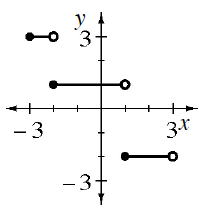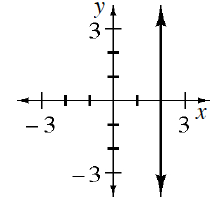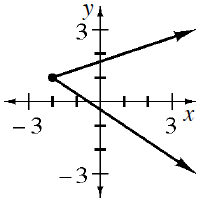### Home > CCA2 > Chapter 1 > Lesson 1.2.3 > Problem1-109

1-109.

For each graph below, what are the domain and range?

See the Math Notes box in Lesson 1.1.3.

See problem 1-62 for more help.

1.Domain: $−3\le x<3$
Range: $y=−2,1,3$

1.Domain: $x=2$
Range: $−∞

1.